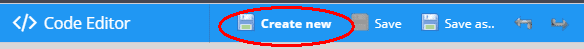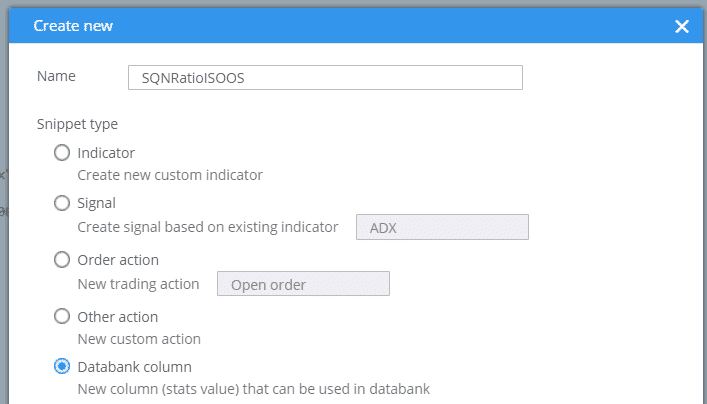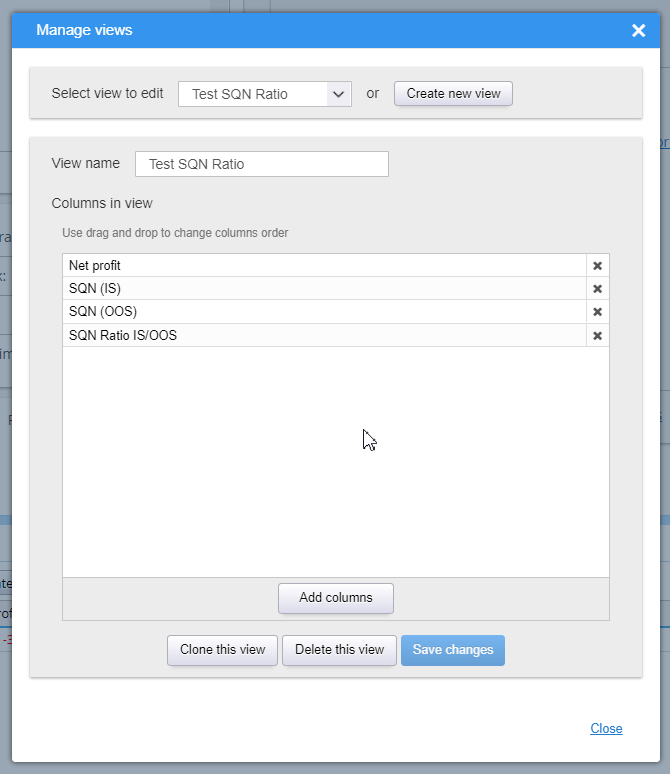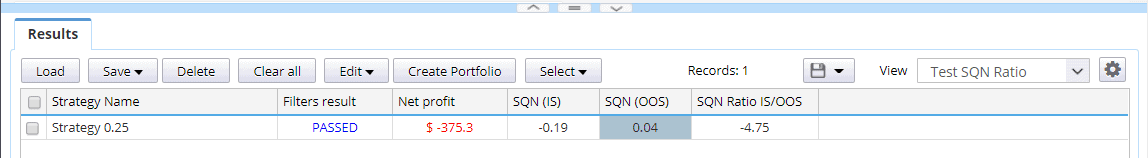# Adding new databank column – special

Author: Mark Fric

March 1st, 2019

Rating:(No Ratings Yet)Loading...

In this example we will be adding new metrics (databank column) that will be computed as ratio of two already computed column values. In our case we want to get ratio of SQN(IS) vs SQN (OOS).

So the formula will be simple:

`SQN IS OOS ratio = SQN (IS) / SQN (OOS)`

We’ll go step by step:

# Create new databank column snippet

Switch to Code editor:then click on Create new button:Set SQNRatioISOOS as a name of new column, and choose Databank column type:This will create a new column snippet with a standard template.

This new snippet doesn’t do much. A new column will be added to databank after you recompile and
restart SQ, but it will display normal Net profit value because this is the example computation in the
standard template.

# Compute the desired value in the snippet

In order for snippet to compute the value we want we need to make a few modifications.

## First, we’ll modify its constructor (first method)

Original code was:

```public SQNRatioISOOS() {
super("SQNRatioISOOS",
DatabankColumn.Decimal2, // value display format
ValueTypes.Maximize, // whether value should be maximized / minimized / approximated to a value
0, // target value if approximation was chosen
0, // average minimum of this value
100); // average maximum of this value

setWidth(80); // defaultcolumn width in pixels

/* If this new column is dependent on some other columns that have to vbe computed first, put them here.
Make sure you don't creat circular dependency, such as A depends on B and B depends on A.
Columns (=stats values) are identified by the name of class)
*/
}```

we’ll change it to:

```public SQNRatioISOOS() {
super("SQN Ratio IS/OOS",
DatabankColumn.Decimal2, // value display format
ValueTypes.Maximize, // whether value should be maximized / minimized / approximated to a value
0, // target value if approximation was chosen
0, // average minimum of this value
100); // average maximum of this value

setWidth(80); // defaultcolumn width in pixels

setTooltip("Ratio of SQN (IS) / SQN (OOS)");
}```

We didn’t do anything special, only specified better name for our snippet and set tooltip help. We arso deleted comments with dependencies we don’t need.

## Next we’ll delete the compute() method

Normally we woudl use this method to compute the snippet value. This method can be used to compute the metrics value from list of trades or as a ratio between some already computed fields.

Unfortunately, in our case we need to compute ratio between the same stats value (SQN) but computed for two different parts of the data – In Sample and Out of Sample.

This cannot be done in compute() method, so we can delete it.

we have to override a special method getValue() that is called when databank grid retrieves value for this cell.

In this method we can retrieve separate stats for IS and OOS part, and from them the values of SQN of IS and OOS part.

```public String getValue(ResultsGroup results, String resultKey, byte direction, byte plType, byte sampleType) throws Exception {
// get stats for IS and OOS part
SQStats statsIS = results.subResult(resultKey).stats(Directions.Both, PlTypes.Money, SampleTypes.InSample);
SQStats statsOOS = results.subResult(resultKey).stats(Directions.Both, PlTypes.Money, SampleTypes.OutOfSample);

double sqnIS = 0, sqnOOS = 0;

// get values of SQN for IS and OOS. We have to make sure that stats objects are not null
if(statsIS != null) sqnIS = statsIS.getDouble("SQN");
if(statsOOS != null) sqnOOS = statsOOS.getDouble("SQN");

// nw compute and return their ratio - note that we use safeDivide because sqnOOS can be also zero
double ratio = SQUtils.safeDivide(sqnIS, sqnOOS);

return String.format("%.2f", ratio);
}```

this is all that is needed. Now snippet will compute value fo this cell as a ratio of already existing values of SQN (IS) and SQN (OOS).

Hit Compile button and you should see the result with successful compilation:Now restart SQ and you should see this new column in available columns. To use it, open Manage view in some databank and create or edit a view:Save, then select this view in your datbank and you should see your new column properly computed:That’s all – we have new databank column snippet.